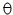Name: ___________________Date:___________________

 Email us to get an instant 20% discount on highly effective K-12 Math & English kwizNET Programs!

High School Mathematics - 2 Introduction to Trigonometry: Degrees and Radians

Introduction
The word 'trigonometry' is derived from the Greek words 'trigon' and 'metron' and it means measuring the sides of a triangle.

Angles
An angle is considered as a figure obtained by rotating a given ray about its initial point. The original rotation of the ray is called the initial side and the final position of the ray after rotation is called the terminal side of the angle. The point of rotation is called the vertex. If the direction of rotation is anti-clockwise, the angle is positive and if the direction of rotation is clockwise, then the angle is negative.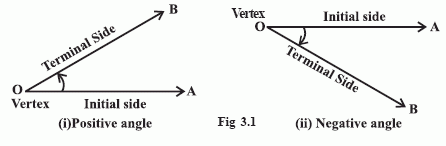Degree Measure

• The angle that measures one degree (1o) is (1/360th) of a revolution.
• One sixtieth of a degree is called a minute.(1l)
• One sixtieth of a minute is called a second. (1ll)
• Some of the angles whose measures are 180o, 2700, 420o, -30o, -420o as shown below.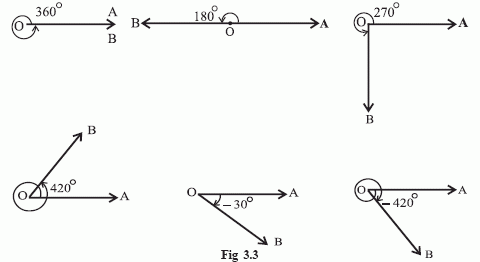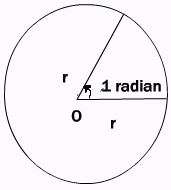• This is another unit for measurement of an angle, called the radian measure. In this system, the unit of measurement is a radian.
• An angle with its vertex at the centre of a circle which intercepts an arc equal in length to the radius of the circleis said to have a measure of 1 radian.
• We know that the circumference, S of a circle of radius r is 2pr. Thus one complete revolution subtends an angle of 2p/r = 2p radian.
It is well known that equal arcs of a circle subtend equal angles at the centre. Since an arc of length r subtends an angle whose measure is l radian, therefore an arc of length l will subtend an angle whose measure is l/r radians. Thus, if in a circle of radius r, an arc of length l subtends an angle theta radians at the centre, we have
q = l/r

Relation between degrees and radians
Since a circle subtends at the centre an angle whose measure is 2p nits in radians and it is 360 units in degrees, it follows that
2p radian = 360 degrees
p radian = 180 degrees.
Using the above relation we get
1 radian = 180 degrees/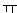= 57o16l
Also 1o =/180 radian = 0.01746 approximately.

 Degree-Radian Conversion Radian-Degree Conversion 1800=p 900=p/2 450=p/4 1370=137p/180 p/3 = 600 p/6 = 300 7p/3 = 4200 3p/4 = 1350

Example: Find the radius of the circle in which a central angle of 45 degrees intercepts an arc of 187 cm.
Solution: Here l= 187 cm and angle = 45/180 =/4
Hence by r = l/